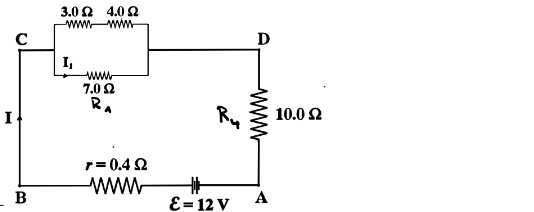# Problem: Four resistors are connected as shown in the figure to a battery with emf ε = 12 V   and internal resistance r = 0.4Ω. What is the equivalent resistance of the four resistors, i.e., not including the internal resistance of the battery?

84% (10 ratings)
###### Problem Details

Four resistors are connected as shown in the figure to a battery with emf ε = 12 V   and internal resistance = 0.4Ω. What is the equivalent resistance of the four resistors, i.e., not including the internal resistance of the battery?Frequently Asked Questions

What scientific concept do you need to know in order to solve this problem?

Our tutors have indicated that to solve this problem you will need to apply the Combining Resistors in Series & Parallel concept. You can view video lessons to learn Combining Resistors in Series & Parallel. Or if you need more Combining Resistors in Series & Parallel practice, you can also practice Combining Resistors in Series & Parallel practice problems.

How long does this problem take to solve?

Our expert Physics tutor, Juan took 2 minutes and 25 seconds to solve this problem. You can follow their steps in the video explanation above.